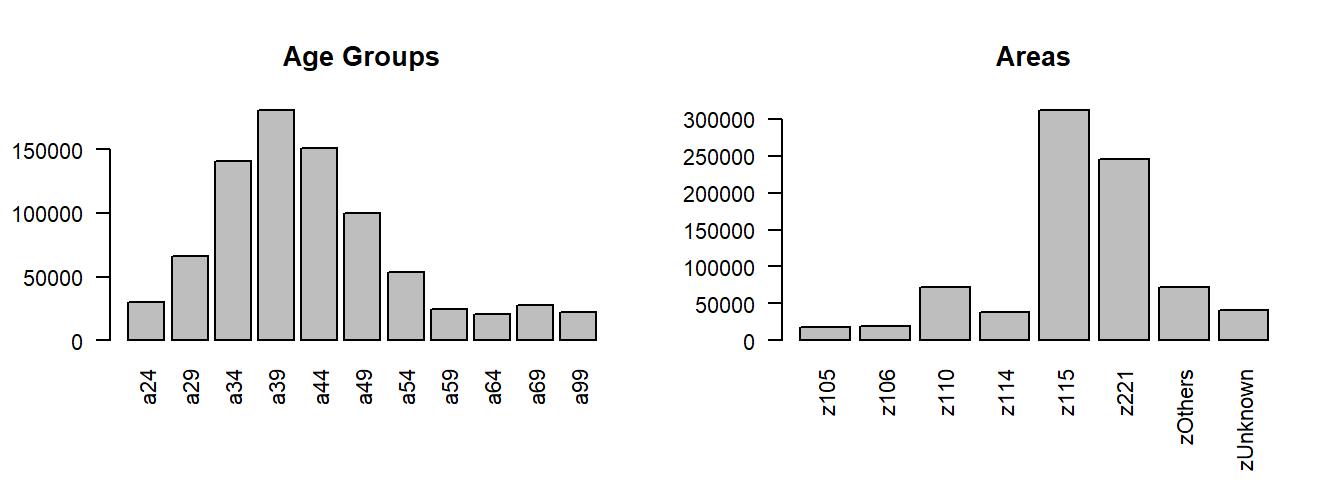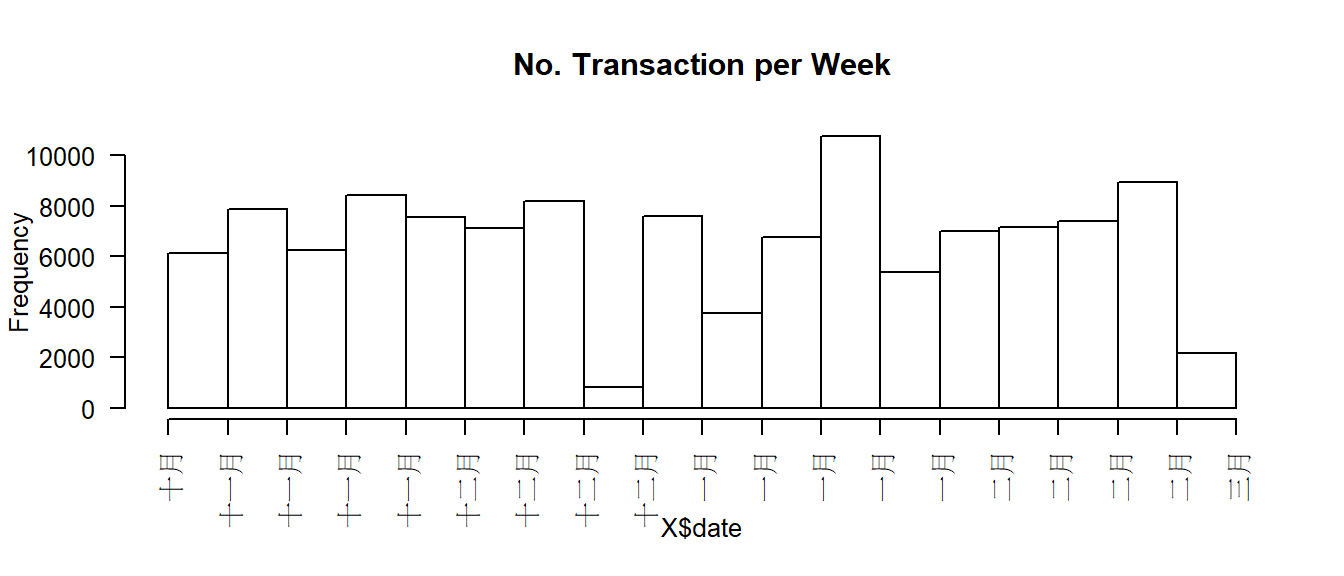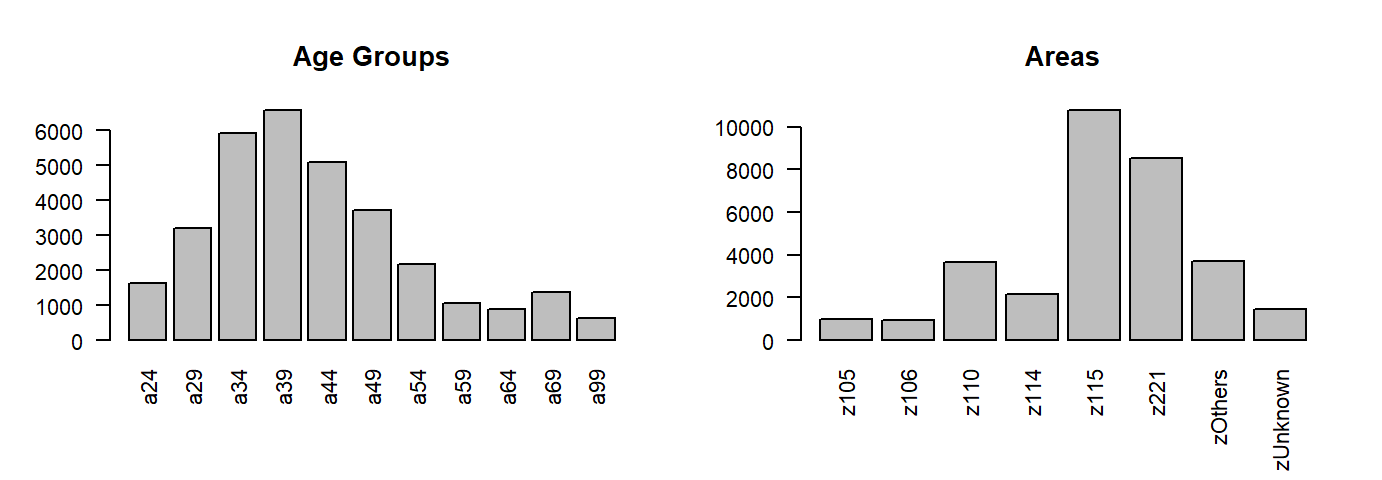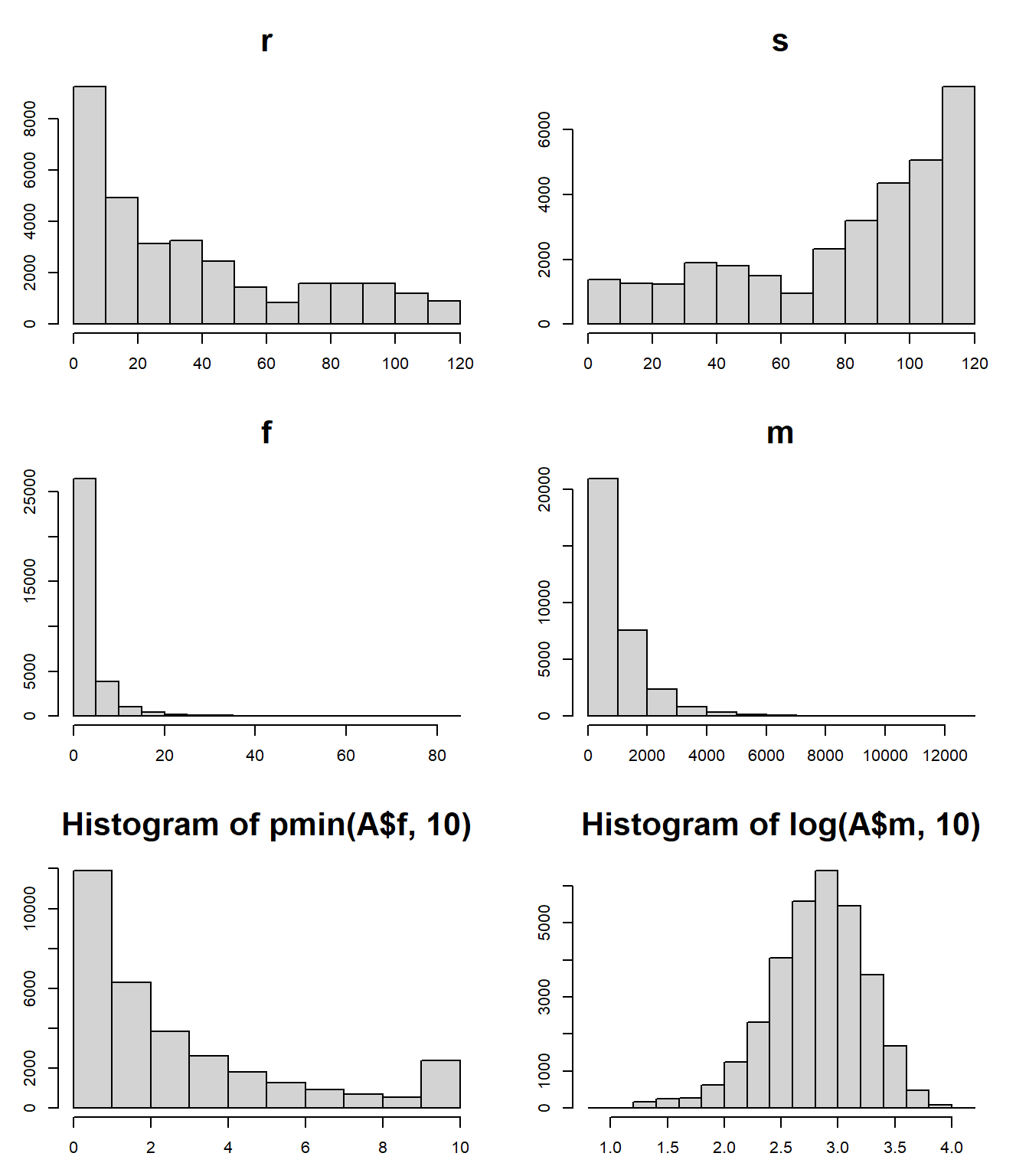Data Preparation : Summarize Data by the Unit of Analysis

• Order Items : Z 817,182
• Orders : X 119,328
• Customers : A 32,241

### 1. Order Items：Z

rm(list=ls(all=T))
pacman::p_load(magrittr, readr, caTools, ggplot2, dplyr, vcd)
Z = read_csv("data/ta_feng_all_months_merged.csv") %>%
data.frame %>% setNames(c(
"date","cust","age","area","cat","prod","qty","cost","price"))
## Rows: 817741 Columns: 9
## -- Column specification --------------------------------------------------------
## Delimiter: ","
## chr (5): TRANSACTION_DT, CUSTOMER_ID, AGE_GROUP, PIN_CODE, PRODUCT_ID
## dbl (4): PRODUCT_SUBCLASS, AMOUNT, ASSET, SALES_PRICE
##
## i Use spec() to retrieve the full column specification for this data.
## i Specify the column types or set show_col_types = FALSE to quiet this message.
nrow(Z)
##  817741
##### 1.2 Data Conversion
Z$date = as.Date(Z$date, format="%m/%d/%Y")
par(cex=0.8)
table(Z$area,useNA='ifany') %>% barplot(main="Areas", las=2)##### 1.4 Handle Outliers # Quantile of Variables sapply(Z[,7:9], quantile, prob=c(.99, .999, .9995)) ## qty cost price ## 99% 6 858.0 1014.00 ## 99.9% 14 2722.0 3135.82 ## 99.95% 24 3799.3 3999.00 # Remove Outliers Z = subset(Z, qty<=24 & cost<=3800 & price<=4000) nrow(Z)  ##  817182 ### 2. Orders, Transaction Records：X Treat every distinct customer-date combinations as a transaction (order) Z$tid = group_indices(Z, date, cust) # assign transaction id
## Warning: The ... argument of group_keys() is deprecated as of dplyr 1.0.0.
## Please group_by() first
## This warning is displayed once every 8 hours.
## Call lifecycle::last_warnings() to see where this warning was generated.

n_distinct Number of distinct …..

# No. cust, cat, prod, tid
sapply(Z[c("cust","cat","prod","tid")], n_distinct)
##   cust    cat   prod    tid
##  32256   2007  23789 119422
##### 2.1 Summarise by tid transaction id
X = Z %>% group_by(tid) %>% summarise(
date = min(date),          # date
cust = min(cust),          # customer id
age = min(age),            # age code
area = min(area),          # zip code
items = n(),               # no. items
pieces = sum(qty),         # total quantity
total = sum(price),        # total revenue
gross = sum(price - cost)  # total gross margin
) %>% data.frame
nrow(X) # 119422 
##  119422
##### Handle Outliers
# Check Quantile & Remove Outliers
sapply(X[,6:9], quantile, prob=c(.999, .9995, .9999))
##        items   pieces     total    gross
## 99.9%     54  81.0000  9009.579 1824.737
## 99.95%    62  94.2895 10611.579 2179.817
## 99.99%    82 133.0000 16044.401 3226.548
# Remove Outliers
X = subset(X, items<=62 & pieces<95 & total<16000) # 119328
##### 交易摘要
summary(X)    
##       tid              date                cust               age
##  Min.   :     1   Min.   :2000-11-01   Length:119328      Length:119328
##  1st Qu.: 29855   1st Qu.:2000-11-29   Class :character   Class :character
##  Median : 59705   Median :2001-01-01   Mode  :character   Mode  :character
##  Mean   : 59712   Mean   :2000-12-31
##  3rd Qu.: 89581   3rd Qu.:2001-02-02
##  Max.   :119422   Max.   :2001-02-28
##      area               items            pieces           total
##  Length:119328      Min.   : 1.000   Min.   : 1.000   Min.   :    5.0
##  Class :character   1st Qu.: 2.000   1st Qu.: 3.000   1st Qu.:  227.0
##  Mode  :character   Median : 5.000   Median : 6.000   Median :  510.0
##                     Mean   : 6.802   Mean   : 9.222   Mean   :  851.6
##                     3rd Qu.: 9.000   3rd Qu.:12.000   3rd Qu.: 1080.0
##                     Max.   :62.000   Max.   :94.000   Max.   :15345.0
##      gross
##  Min.   :-1645.0
##  1st Qu.:   21.0
##  Median :   68.0
##  Mean   :  130.9
##  3rd Qu.:  168.0
##  Max.   : 3389.0
##### 每周交易次數
par(cex=0.8)
hist(X$date, "weeks", freq=T, las=2, main="No. Transaction per Week")### 3. Customer：A ##### Summarise by Customer d0 = max(X$date) + 1
A = X %>% mutate(
days = as.integer(difftime(d0, date, units="days"))
) %>% group_by(cust) %>% summarise(
r = min(days),      # recency
s = max(days),      # seniority
f = n(),            # frequency
m = mean(total),    # monetary
rev = sum(total),   # total revenue contribution
raw = sum(gross),   # total gross profit contribution
age = min(age),     # age group
area = min(area),   # area code
) %>% data.frame
nrow(A) # 32241
##  32241
par(mfrow=c(1,2),cex=0.7)
table(A$age, useNA='ifany') %>% barplot(main="Age Groups",las=2) table(A$area, useNA='ifany') %>% barplot(main="Areas",las=2)par(mfrow=c(3,2), mar=c(3,3,4,2))
for(x in c('r','s','f','m'))
hist(A[,x],freq=T,main=x,xlab="",ylab="",cex.main=2)
hist(pmin(A$f,10),0:10,freq=T,xlab="",ylab="",cex.main=2) hist(log(A$m,10),freq=T,xlab="",ylab="",cex.main=2)##### 顧客摘要
summary(A) 
##      cust                 r                s                f
##  Length:32241       Min.   :  1.00   Min.   :  1.00   Min.   : 1.000
##  Class :character   1st Qu.:  9.00   1st Qu.: 56.00   1st Qu.: 1.000
##  Mode  :character   Median : 26.00   Median : 92.00   Median : 2.000
##                     Mean   : 37.45   Mean   : 80.78   Mean   : 3.701
##                     3rd Qu.: 60.00   3rd Qu.:110.00   3rd Qu.: 4.000
##                     Max.   :120.00   Max.   :120.00   Max.   :85.000
##        m                rev              raw              age
##  Min.   :    8.0   Min.   :     8   Min.   : -784.0   Length:32241
##  1st Qu.:  365.0   1st Qu.:   707   1st Qu.:   75.0   Class :character
##  Median :  705.7   Median :  1750   Median :  241.0   Mode  :character
##  Mean   :  993.1   Mean   :  3152   Mean   :  484.6
##  3rd Qu.: 1291.0   3rd Qu.:  3968   3rd Qu.:  612.0
##  Max.   :12636.0   Max.   :127686   Max.   :20273.0
##      area
##  Length:32241
##  Class :character
##  Mode  :character
##
##
## 
##### Check & Save
is.na(Z) %>% colSums
##  date  cust   age  area   cat  prod   qty  cost price   tid
##     0     0     0     0     0     0     0     0     0     0
is.na(X) %>% colSums
##    tid   date   cust    age   area  items pieces  total  gross
##      0      0      0      0      0      0      0      0      0
is.na(A) %>% colSums
## cust    r    s    f    m  rev  raw  age area
##    0    0    0    0    0    0    0    0    0
A0 = A; X0 = X; Z0 = Z
save(Z0, X0, A0, file="data/tf0.rdata")

💡 Common Exploring Questions：
§ How consuming behavior varies by age and area groups?
§ Between weekday and weekend, are consumers behave in the same ways?
§ What are the best selling products and categories?
§ Compare the revenue and profit contribution by product (categories).
§ Segmentation, Define Groups of Customers in different ways
§ Compare the characteristics among the groups
§ Targeting, Focus on some group(s) of customers
§ Objective, Set the goal(s) for improvement